14Motion of a charged particle in the magnetic field
Оценка 4.8

# 14Motion of a charged particle in the magnetic field

Оценка 4.8
pptx
07.05.2020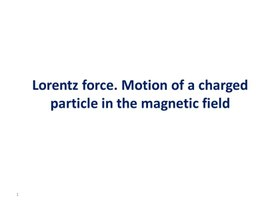14Motion of a charged particle in the magnetic field.pptx

## Lorentz force. Motion of a charged particle in the magnetic field 1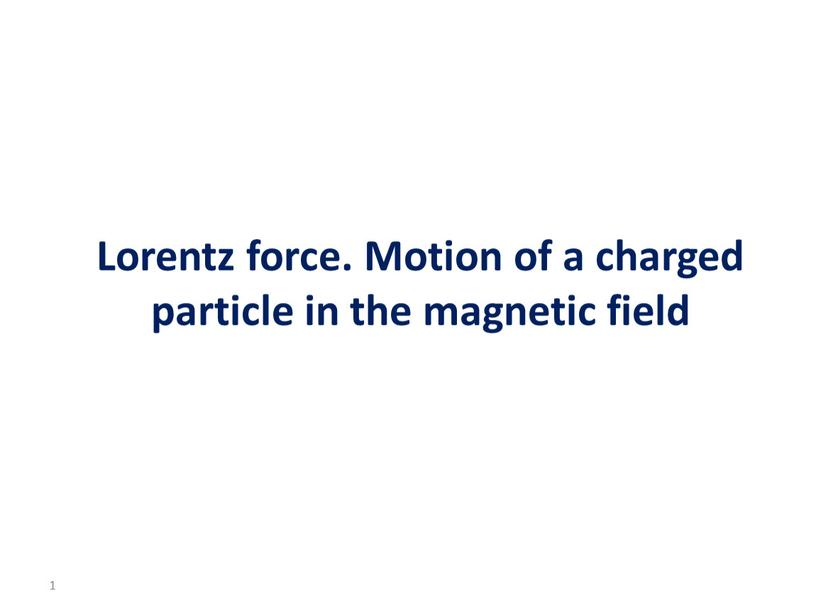Lorentz force. Motion of a charged particle in the magnetic field

1

## Learning objective To investigate the effect of a magnetic field on moving charged particles; 2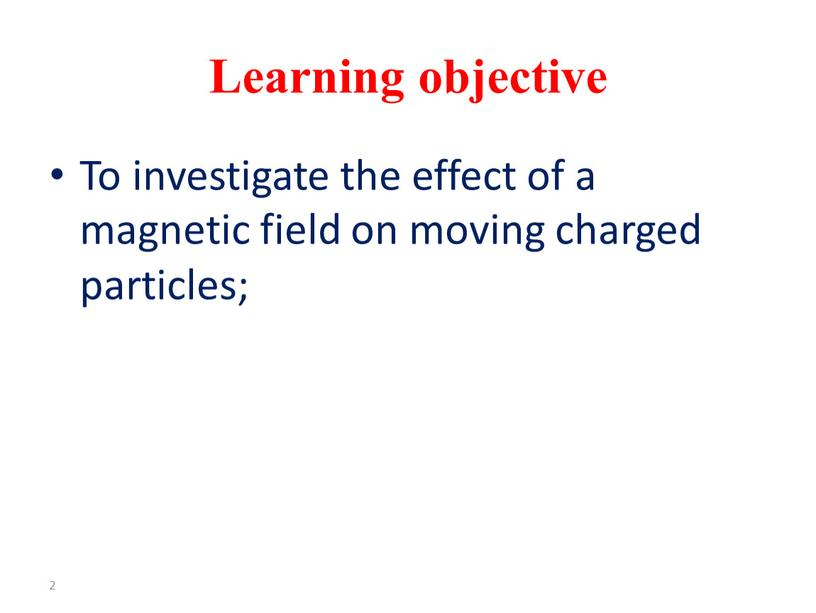Learning objective

To investigate the effect of a magnetic field on moving charged particles;

2

Thompson’s experiment

In 1897 JJ Thomson set out to prove that cathode rays originating from a heated cathode (electron gun), were actually a stream of small negatively charged particles called electrons.
Electrons are accelerated from the cathode.
They are deflected by electric and magnetic fields.
The beam of electrons strikes a fluorescent screen.
e/m was measured.

The charge-to-mass ratio of an electron

Since the electrons are moving in a circle, there must be centripetal force
Question: Which force is acting as the centripetal force?
Therefore, since FM = FC
We can equate the equations and solve for e/m
Task: Try to derive an expression
for e/m.

Solution

Therefore,  Bqv = mv2/r
Re-arranging the terms,
q/m = v/Br
Question: Which variable is hard to
measure?

## Answer Velocity However we get around this using the electron gun equation: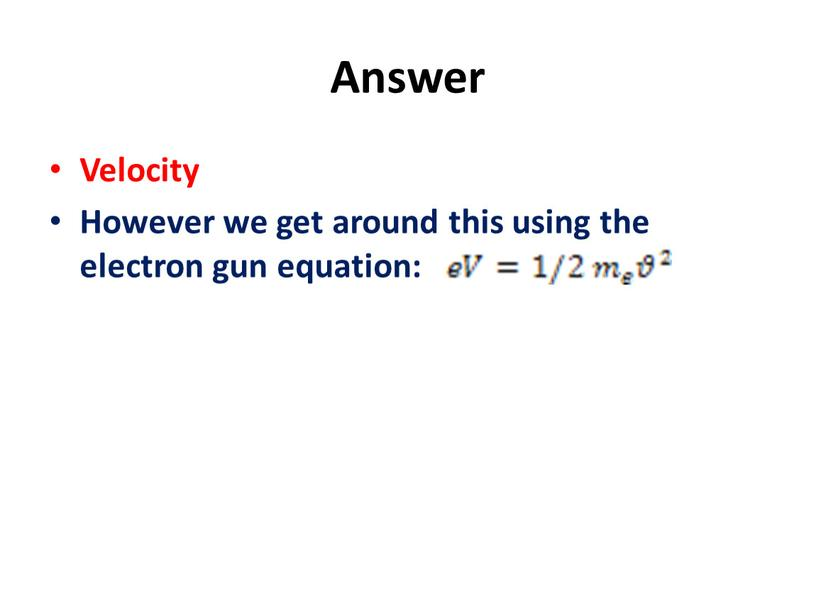Velocity
However we get around this using the electron gun equation:

## The ratio e/m for an electron – its specific charge – may be determined using a fine-beam tube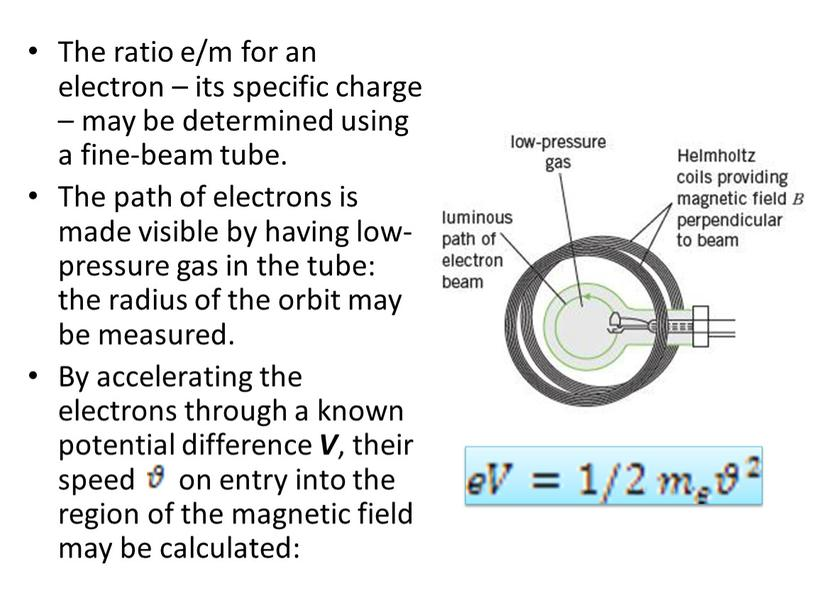The ratio e/m for an electron – its specific charge – may be determined using a fine-beam tube.
The path of electrons is made visible by having low-pressure gas in the tube: the radius of the orbit may be measured.
By accelerating the electrons through a known potential difference V, their speed on entry into the region of the magnetic field may be calculated:

## The magnetic field is provided by a pair of current-carrying coils (Helmholtz coils,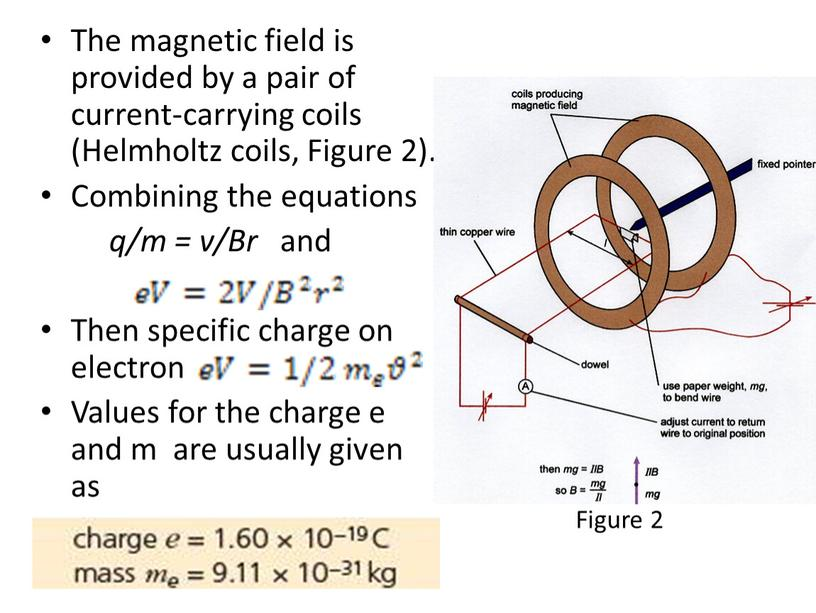The magnetic field is provided by a pair of current-carrying coils (Helmholtz coils, Figure 2).
Combining the equations
q/m = v/Br and

Then specific charge on electron
Values for the charge e and m are usually given as

Figure 2

## Velocity Selector Used when all the particles need to move with the same velocity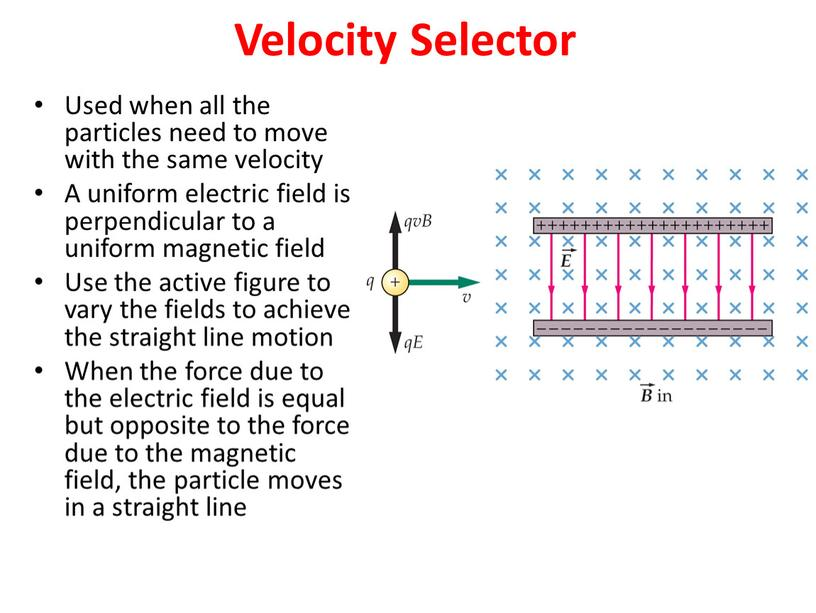Velocity Selector

Used when all the particles need to move with the same velocity
A uniform electric field is perpendicular to a uniform magnetic field
Use the active figure to vary the fields to achieve the straight line motion
When the force due to the electric field is equal but opposite to the force due to the magnetic field, the particle moves in a straight line

## Net force: The forces balance if the speed of the particle is related to the field strengths by qvB = qE v =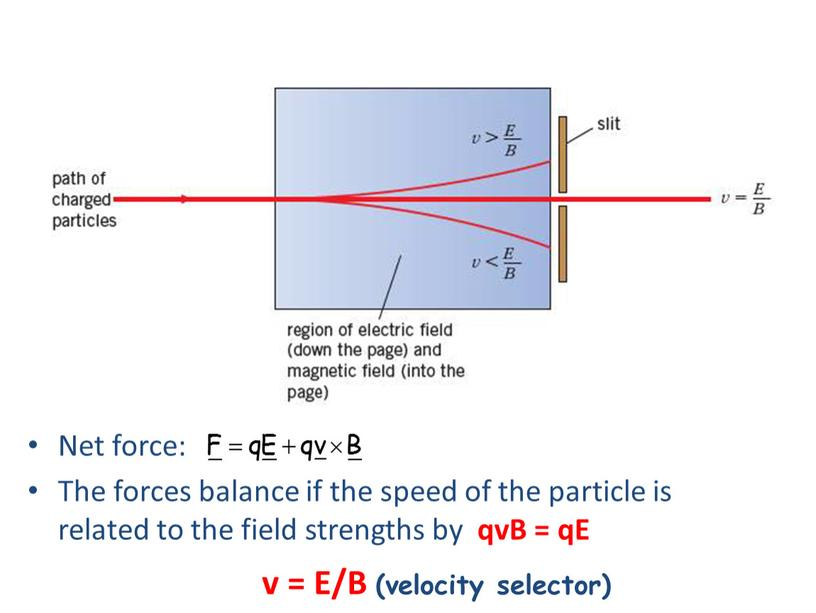Net force:
The forces balance if the speed of the particle is related to the field strengths by qvB = qE

v = E/B (velocity selector)

## Mass Spectrometer A mass spectrometer separates ions according to their mass-to-charge ratio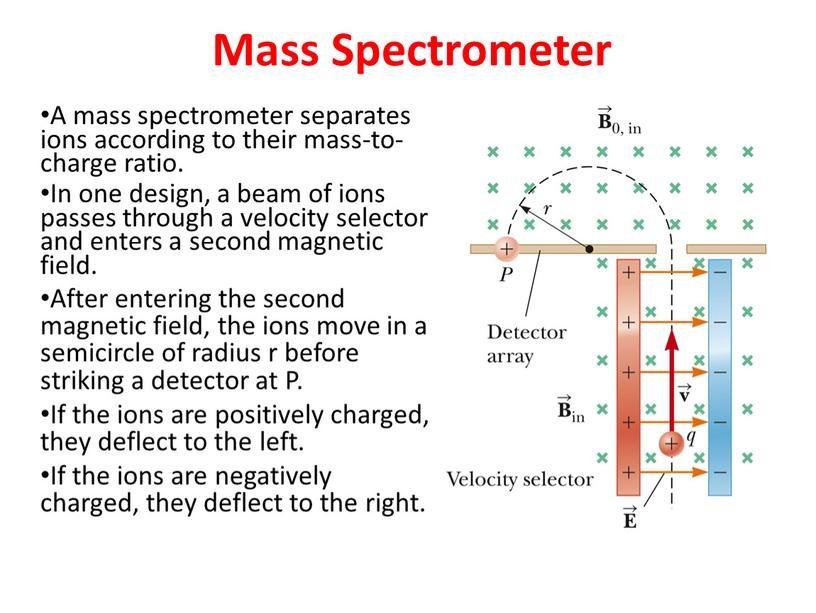Mass Spectrometer

A mass spectrometer separates ions according to their mass-to-charge ratio.
In one design, a beam of ions passes through a velocity selector and enters a second magnetic field.
After entering the second magnetic field, the ions move in a semicircle of radius r before striking a detector at P.
If the ions are positively charged, they deflect to the left.
If the ions are negatively charged, they deflect to the right.

## The mass to charge (m/q) ratio can be determined by measuring the radius of curvature and knowing the magnetic and electric field magnitudes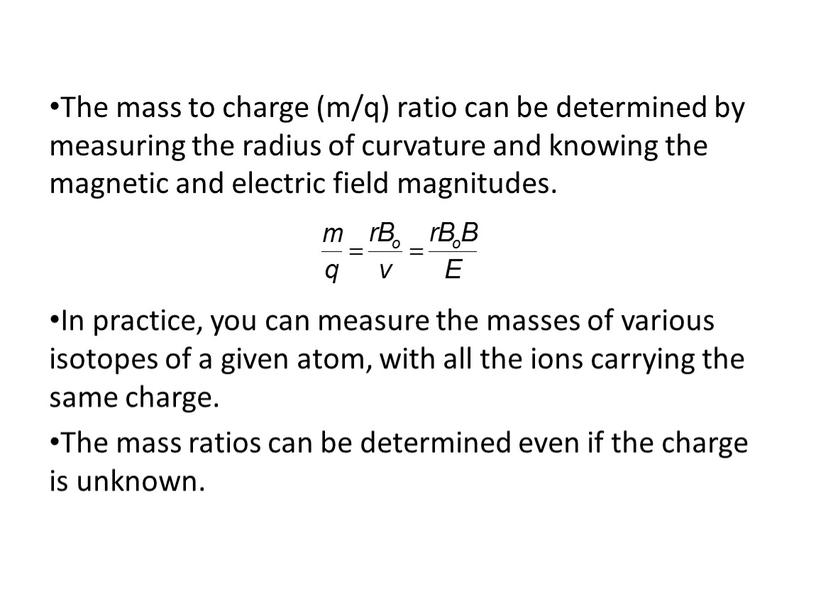The mass to charge (m/q) ratio can be determined by measuring the radius of curvature and knowing the magnetic and electric field magnitudes.

In practice, you can measure the masses of various isotopes of a given atom, with all the ions carrying the same charge.
The mass ratios can be determined even if the charge is unknown.

Reflection

What has been learned
What remained unclear
What is necessary to work on

Скачать файл